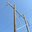# Get area interchange from 230kV lines onlyAs the title suggests, I'm looking for a method to find the area interchange on the 230kV lines.

When I enter ties area into the PSSE command prompt, and pass the required area number (call it 987 for this example), I receive a print out which looks something like the following:

   TO AREA  123   ABC
X---- FROM AREA BUS ----X   X----- TO AREA BUS -----X
BUS# X-- NAME --X BASKV     BUS# X-- NAME --X BASKV  CKT      MW      MVAR
987123 BUS1        230.00*  123123 BUS4        230.00   1      13.9    -25.7
987456 BUS2        230.00*  123456 BUS5        230.00   1     110.7    -14.5
987789 BUS3        115.00*  123789 BUS6        115.00   1     -20.7     -3.0
TOTAL FROM AREA 987 TO AREA 123                                -0.7    -48.3


Now, here's my question.

I know that I can use a command such as psspy.aritoj(987, 123). This will give me (-0.7 - 48.3j), a complex value representing the total net interchange between these areas. This is fine, and I use this at one point in my code.

The problem is that I am also interested in the interchange on the 230kV lines only: the value (124.6 - 40.2j) in this example. (If it makes a difference, all that I need is the real portion of the value, I do not need the entire complex number)

I've been searching around the API and can't seem to find anything to get this value. (it cannot simply be printed to the screen as the ties command does; I need it to be stored in a variable as well as it is to be used elsewhere in the script).

Is there any command which will get me this value?

Thanks for any help.

edit retag close merge delete

Sort by » oldest newest most votedMy solution ended up using the initie command with the relevant area, and then using nxttie() to loop through each tie. First I would check if the bus was in the required area (123 in the example), then I would then check the bus using busdat to see if it was a 230 kV line. If it was, I made sure that I had the correct metered end, and then I would add the real part of brnflo to my running total, and this would give me the total in the end. Example code:

myArea = 987
toArea = 123
kV = 230.0
interchange230 = 0

ierr = psspy.initie(myArea)
ierr, ibus, jbus, ickt = psspy.nxttie()

while ierr == 0:
ierr, ival = psspy.busint(jbus, 'AREA')
if (ierr == 0) and (ival == toArea):
ierr, rval = busdat(jbus, 'BASE')
if (ierr == 0) and (rval == kV):
ierr, ival = psspy.brnint(ibus, jbus, ickt, 'METER')
if (ival == ibus):
ierr, cmpval = psspy.brnflo(ibus, jbus, ickt)
if (ierr == 0):
interchange230 += cmpval.real
else:
ierr, cmpval = psspy.brnflo(jbus, ibus, ickt)
if ierr == 0:
i       interchange230 += -(cmpval.real)

moremaccartm,

I think the following may get the job done for you:

psspy.bsysinit(0)
psspy.bsys(0,1,[229,231],<count of areas>,[<areas>],0,[],0,[],0,[])
psspy.ties(0,0)


The above code initializes subsystem 0, containing only the 229-231 kV system in the areas you specify. Then it uses the ties API to return the flows on all the area tie lines, across all areas in the specified subsystem.

Hopefully this works. Good luck.

EDIT: Oops, you need variables. I guess the best you can do is direct the output from the above code into a file, and do some processing on the file to get what you need. PSSe output files are generally pretty simple to process with python.

more